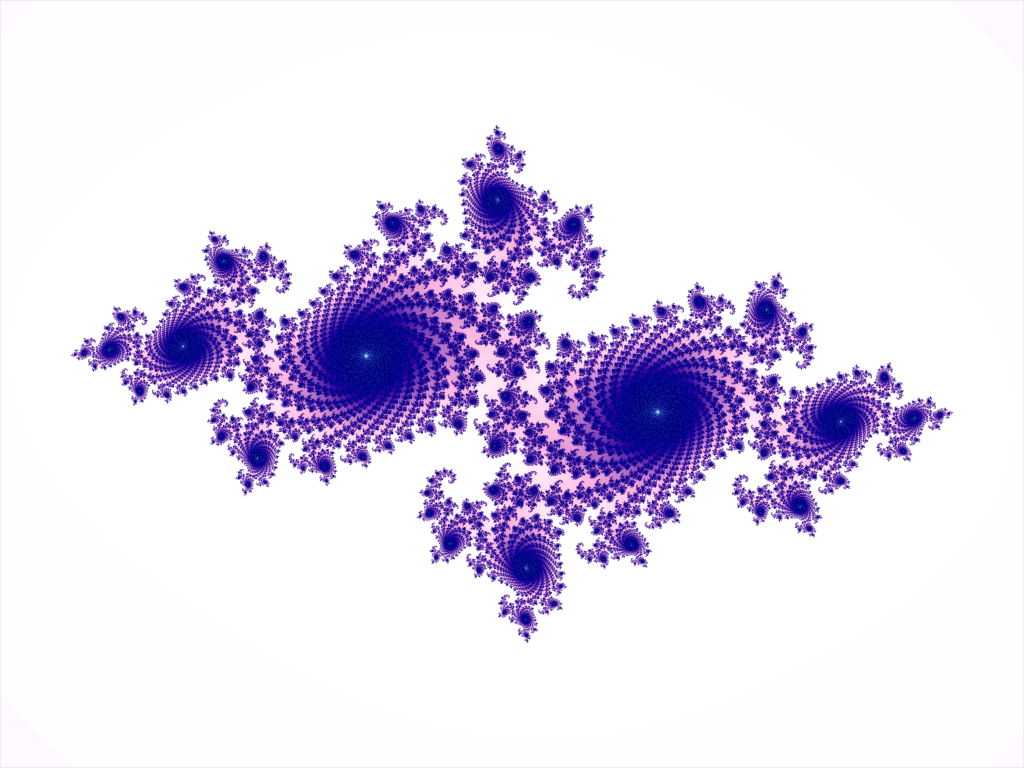# Fractals: Why am I Repeating Myself?

#### by Logan DonougheYou may have seen them on college dorm room walls or on your plate of cauliflower… you may know them when you see them, but what are fractals, really?

Nearly all man-made fractals are recursively generated.1 This is typically done in one of two ways: iteratively removing or changing parts of an existing image or computing the image of a function at each point of an image to the desired resolution.

There are two criteria for determining whether or not an image is a fractal. The more straightforward criterion is that a fractal must be self-similar.

It is easiest to see self-similarity at work in iterative replacement fractals. These fractals are generated by repeatedly replacing or changing aspects of the previous iteration. For example, the creation of The Sierpinski Triangle via recursive replacement can be seen in Figure 1. Each triangle remaining in the set (the dark ones) has a triangle constructed from the points bisecting it’s edges removed from the set creating 3 separate, smaller triangles in the set in place of the original triangle.

The second criterion is the “soft” criterion that it’s Hausdorff Dimension should be greater than it’s geometric or “typical” dimension. Unfortunately, a deep discussion of Hausdorff Dimension is beyond the scope of this brief overview. However, it is worth noting that for most images, the Hausdorff Dimension is equal to the Geometric Dimension when both exist. The Hausdorff Dimension is created through the use of ball coverings of the measured surface.

The notion of a fractal as well as this criterion were popularized, if not created, by Benoit Mandelbrot. The Mandelbrot Set (the “classic” fractal which appears in Figure 2), accordingly, is named for its discoverer.

The Mandelbrot is characterized by the recursive function:

zn+1= zn2+ c

where z0  is the starting complex point on a plane.

A point z0 is in the Mandelbrot Set if limn -> ∞ |zn| is bounded. For this particular function, this is the same as saying that for all n, |zn| < 2

Note that most images of the Mandelbrot Set are colored in some manner (not simply portrayed in black and white). The addition of color is usually done by attributing a color to the iteration in which each sequence from associated with points outside of the set diverge (become a distance greater than 2 from the origin of the complex plane).

The Julia Set” (viewable as Figure 3 and as the featured image of this post) is the most interesting case resulting from taking The Julia Set of a function. The Julia Set is the set of points where a function from the complex numbers to the complex numbers behaves erratically (i.e. it’s behavior at one point is not possible to generalize from it’s surrounding points). The function used when one is talking out of the context of a specific function about “The Julia Set” is nearly always referring to the Julia Set of the same function that generates The Mandelbrot Set:

f(z)= z2+ c

The obvious application of fractals is the direct use of images of fractals as art. Besides this, fractals have found their way into the generation of terrain and other elements in video games. For infinitely explorable worlds, content is usually generated via algorithm due to the fact that creating infinite content by hand is impossible on finite time scales. These algorithms often generate fractals or fractalesque terrain and environments due to their inherent complexity and intricacy which lend a degree of interest and unpredictability to otherwise drab and overly repetitive landscapes.

Fractals are often the go to subject to convince skeptics that “math is cool” yet few seem to bother to look into their properties and construction. Although this is just a brief overview, it is my hope that it inspires some further study and thus understanding.

1If you wish to learn how naturally occurring fractals are created, I advise you to look into the forces that affect the creation of whichever phenomenon you wish to know about. In one word, science.

The thumbnail image (which is also Figure 3) is in the public domain courtesy of it’s creator: Eequor

Figure 1 is also in the public domain as well as Figure 2 (courtesy of it’s creator Adam Majewski)